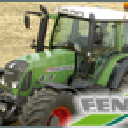• non_pushable1 = bit1 = 2 (terrain delta)
non_pushable2 = bit2 = 4
static_world1 = bit3 = 8 (terrain delta)
static_world2 = bit4 = 16 = 0x10
ai_blocking = bit5 = 32 = 0x20

tractors = bit6 = 64 = 0x40
combines = bit7 = 128 = 0x80
trailers = bit8 = 256 = 0x100

dynamic_objects = bit12 = 4096 = 0x1000

dynamic_objects_machines = bit13 = 8192 = 0x2000 flag for all machines, to make them collide

ground_tip_blocking = bit19 = 524288 = hex 80000

trigger_player = bit20 = 1048576 = hex 100000
trigger_tractors = bit21 = 2097152 = hex 200000
trigger_combines = bit22 = 4194304 = hex 400000
trigger_fillables = bit23 = 8388608 = hex 800000 (trailers, sprayers, sowingmachine)

trigger_dynamic_objects = bit24 = 16777216 = hex 1000000

trigger_trafficVehicles = bit25 = 33554432 = hex 2000000

trigger_cutters = bit26 = 67108864 = hex 4000000

trigger_fork = bit27 = 134217728 = hex 8000000 (bales, pallets, ..)

kinematic_objects_without_collision = bit30 = 1073741824 = hex 40000000

bits for terrain delta= 1,3 = 10 = 0xA

bits for tractors= 1,6,13,21 = 2105410 = 0x202042
bits for combines= 1,7,13,22 = 4202626 = 0x402082
bits for fillables= 1,8,13,23 = 8397058 = 0x802102

bits for tools = 1,13 = 8194

bits for tools without terrain delta collision = 2,13 = 8196

bits for terrain = all but triggers and ai_blocking (first 20 bits)
= 11111111111111011111 = 1048543 = 0xFFFDF

bits for pushable dynamic objects = 3,12,13,24 = 16789512 = 0x1003008

100000000000000011110
111111111111111111111
1111010000110011101001

bits for cutters = 1,12,13,26 = 67121154 = 0x4003002

11111111111111111111111111111111
10 non_pushable1
100 non_pushable2
1000 static_world1
10000 static_world2

1000000000000 dynamic_objects
100000000000000000000 trigger_player

1000000011110 "cct move" = 4120
100000000000000010110 "cct create" = 1048598 = 0x100016
1000000000001000000001000

1000000000000000000000 trigger_tractors
10000000000000000000000 trigger_combines
100000000000000000000000 trigger_trailers
1000000000000000000000000 trigger_dynamic_objects

10000000000000 dynamic_objects_machines

1000000010000001000010 "tractors"
10000000010000010000010 "combines"
100000000010000100000010 "trailers"
1000000000011000000001000 "pushable dynamic objects"

## Jetzt mitmachen!

Sie haben noch kein Benutzerkonto auf unserer Seite? Registrieren Sie sich kostenlos und nehmen Sie an unserer Community teil!